Journal DetailsFormat
Journal
eISSN
2354-0192
First Published
28 Dec 2018
Publication timeframe
1 time per year
Languages
EnglishOpen Access

# Transient response of “Brezina concrete arch dam”–“Reservoir water”–“Rock foundation” system

###### Accepted: 14 Nov 2021
Journal DetailsFormat
Journal
eISSN
2354-0192
First Published
28 Dec 2018
Publication timeframe
1 time per year
Languages
English

In this paper, transient responses of the Brezina concrete arch dam, located in the west of Algeria, are determined using the finite elements commercial package, Ansys. A 3D model has been created to study the rock–fluid–structure interaction phenomenon. The foundation rock is modeled as a mass rock using solid finite elements, and the reservoir water is modeled using fluid finite element for the 3D model; the length and width of the foundation rock and water reservoir, along the global X and Y axes, are taken to be 150 m and 300 m, respectively, while its depth (or height), along the Z direction, is taken to be 100 m (the total height of the model is thus 160 m considering that the reservoir water height is taken to be 50 m). Coupling equations available in the Ansys code library are used to represent the dam–water and dam–foundation interfaces. It is found that the role of the reservoir water is mixed. In some cases, its presence is like a damper, which means it decreases the range of displacement or stresses, whereas in other cases, it increases or has a negligible effect on these quantities. A notable finding is the magnitude of the calculated stresses, which stands at a very low level, either with or without water. An in-depth review of the literature reveals that the study carried out in this research encompasses several elements of originality, as only very few similar works have been undertaken.

#### Keywords

Introduction

One of the main topics in earthquake engineering is the dynamic analysis of dam–reservoir systems. Research on the dam–foundation interaction problem is available extensively in the literature [1,2,3,4,5,6,7,8].

There are several extensive methods that have been applied in past decades. Numerical procedures that include the interaction between several domains with different properties—concrete dam, foundation rock, water, bottom sediments, and bank of the reservoir—have been developed using the finite element method, the boundary element method, and various combinations of both the methods [9,10,11,12, 21, 22].

Evaluation of the important hydrodynamic forces that develop on the upstream face of a large dam during severe transient excitations has been the subject of numerous studies, starting with Westergaard's classical work in 1933. Westergaard explained the physical behavior of dam–reservoir interaction for 2D coupled systems .

According to the literature, two approaches may be used to model the dam–reservoir interaction phenomenon: the coupling method and the contact elements method .

As part of the comprehensive study undertaken on the dynamic response of the Brezina arch dam, located in El Beyadh, Algeria, and following recent works [14, 19], in this paper, the influence of the reservoir water on the transient response of the Brezina arch dam is investigated. This article is the continuity of Berrabah et al. , which studies the modal behavior of the same concrete dam, taking into account the fluid–structure interaction phenomenon.

Coupled Fluid–Structure–Foundation Formulation

The discretization of the structural dynamic equation taking into account both the dam and foundation rock subjected to ground motion, using the finite-element approach, can be given as: $Msu¨e+Csu˙e+Ksue=−Msu¨g+QPe$ {M_s}{\ddot u_e} + {C_s}{\dot u_e} + {K_s}{u_e} = - {M_s}{\ddot u_g} + Q{P_e} where Ms is the structural mass; Cs is the damping matrix; Ks is the stiffness matrix; ue is the nodal displacement vector with respect to ground; and QPe represents the nodal force vector associated with the hydrodynamic pressure produced by the reservoir. In addition, üe and üg are the relative nodal acceleration and nodal ground acceleration vectors, respectively. The term Q represents the coupling matrix.

The hydrodynamic pressure equation discretization is given as: $MfP¨e+CfP˙e+KfPe+ρwQT(u¨e+u¨g)=0$ {M_f}{\ddot P_e} + {C_f}{\dot P_e} + {K_f}{P_e} + {\rho _w}{Q^T}\left( {{{\ddot u}_e} + {{\ddot u}_g}} \right) = 0 where Mf is the fluid mass matrix; Cf is the fluid damping matrix; Kf is the fluid stiffness matrix; Pe is the nodal pressure; üe is the relative nodal acceleration; üg is the nodal ground acceleration vector; and ρwQT is the transpose of the coupling matrix.

The assembled form of both Eqs (1) and (2) can be given as: $[ Ms0MfsMf ]{ u¨eP¨e }+[ Cs00Cf ]{ u˙eP˙e }+[ KsKfs0Kf ]{ uepe }={ −Msu¨g−Mfsu¨g }$ \matrix{ {\left[ {\matrix{ {{M_s}} & 0 \cr {{M_{fs}}} & {{M_f}} \cr } } \right]\left\{ {\matrix{ {{{\ddot u}_e}} \cr {{{\ddot P}_e}} \cr } } \right\} + \left[ {\matrix{ {{C_s}} & 0 \cr 0 & {{C_f}} \cr } } \right]\left\{ {\matrix{ {{{\dot u}_e}} \cr {{{\dot P}_e}} \cr } } \right\}} \hfill \cr { + \left[ {\matrix{ {{K_s}} & {{K_{fs}}} \cr 0 & {{K_f}} \cr } } \right]\left\{ {\matrix{ {{u_e}} \cr {{p_e}} \cr } } \right\} = \left\{ {\matrix{ { - {M_s}{{\ddot u}_g}} \cr { - {M_{fs}}{{\ddot u}_g}} \cr } } \right\}} \hfill \cr } where Kfs = − Q and Mfs = ρwQT.

Eq. (3) is the second-order linear differential equation having unsymmetrical matrices and it can be solved using direct integration methods.

The 3D “Reservoir Water”–“Concrete Arch Dam”–“Rock Foundation” Finite Element Model and Transient Loadings
Geometric description and finite element model

The object of the present work is the Brezina concrete arch dam, shown in Figure 1. It would be helpful to recall that this article is a continuity of Berrabah et al. , which studies the modal behavior of the same concrete dam taking into account its interaction with “reservoir water” and “rock foundation”. The geometric characteristics of the dam are summarized in Table 1.

Geometrical characteristics of Brezina dam

High (m) Arch length (m) Thickness (m)
60 78.5 At crest: 5
At foundation level: 36.3

The dam–reservoir interaction system is investigated using the finite element commercial package, Ansys, with a mapped meshing  3D finite element model.

In order to determine the fineness of meshing , a sensitivity study was performed with respect to the modal and static responses.

The 3D model features 16,252 quadratic solid elements (SOLID186) and 3,000 fluid elements (FLUID80).

It is important to note that coupling equations are used to model the fluid – “rock foundation” and/or fluid–“concrete dam” contact; however, for the “concrete dam”–“rock foundation” interface, “perfect contact” available in the Ansys code is used to ensure continuity and linearity.

Material description

Table 2 summarizes the material properties of the Brezina concrete arch dam, rock–foundation and reservoir water.

Material properties of Brezina arch dam, foundation rock, and reservoir water

Material Young's modulus (N/m2) Poisson's ratio Density (kg/m3)
Concrete dam 28.5e+09 0.2 2,500
Foundation rock 14.5e+09 0.25 2,100
Reservoir water Compressibility modulus (N/m2) Viscosity 0.49 1,000
2.068E+09 0.001
Boundary conditions (BC)

As shown in Figure 2, for the 3D model, the length and width of the foundation rock and water reservoir, along the global X and Y axes, are taken to be 150 m and 300 m, respectively, while its depth (or height), along the Z direction, is taken to be 100 m (the total height of the model is thus 160 m, considering that the reservoir water height is taken to be 50 m). These sizes are sufficiently large as to ensure that the applied BCs do not affect the transient responses of the dam. The size of 150 m is equal to 2.5 times the dam's height, 60 m; taking a factor of 2.5 is a common practice to ensure a good representation of the foundation rock and water reservoir (the study by Sani and Lotfi can be mentioned as an example ).

It is important to note that both rock foundation and reservoir water extensions are chosen after doing sensitivity analysis of the dam behavior via these two parameters. For this reason, an extension of both rock foundation and water reservoir was taken equal first as 50 m and a transient analysis was performed and results were extracted. Then these extensions were increased until the convergence of the extracted results could be obtained (the above extension values are judged as sufficient). At these extension limits, fixed supports are applied. So, at these limits, no radiation damping is present.

It is also worth mentioning that wave propagation is not taken into account, but this can be used as a perspective.

The “reservoir water”–“concrete dam” and “reservoir water”–“rock foundation” coupling is obtained as follows: at an interface, the displacement of the nodes belonging to the “reservoir water” and that of the nodes belonging to the “concrete dam” or “foundation rock” are coupled in the normal direction of the interface. The other two displacement components of these nodes, i.e., the displacement in the tangential and vertical directions, are left free (not coupled). In the studies by Fok and Chopra , Tan and Chopra , and Sani and Lotfi , which employ the Eulerian approach, the coupling is made between the displacements and pressures resulting in the interaction matrix. In this study the Lagrangian approach is employed; the reference configuration of the continuum, solid, or fluid, is the undeformed state; and the behavior of both the fluid and structure is expressed in terms of the displacement .

Two kinds of static loadings are considered in the present article: the self-weight of the dam (or the dam–rock system) and the hydrostatic pressure in the case of the full “reservoir water” system.

To perform transient analyses for the “reservoir water”–“concrete dam”–“foundation rock” model, three synthetic earthquake records (accelerograms) have been generated . Figure 3 depicts the accelerogram in the X direction. In the present study, analyses are carried out using this X-direction accelerogram only. It would be helpful to recall that the X direction is one which is perpendicular to the “concrete dam” crest (i.e., along the longitudinal section of the “reservoir water”). It is important to note that in this article the earthquake record is applied uniformly at the model base, which means that the spatial variability of the earthquake record is not taken into account. For all the analyses performed, the damping coefficient is set at 5% (with respect to the critical damping) by means of Rayleigh damping.

Integration scheme

As mentioned in Berrabah et al. , the accuracy of the transient dynamic solution depends on the integration time step (ITS): the smaller the time step, the higher the accuracy. In this study the Newmark method is used (γ = 0.005 [Ansys default value]).

For the Newmark formulation, both the final velocity basic integration equations and the final displacement basic integration equations are expressed as follows: $υ˙1=υ˙0+(1−γ)Δtυ¨0+γΔtυ¨1υ1=υ0+Δtυ˙0+(12−β)Δt2υ¨0+βΔt2υ¨1$ \matrix{ {{{\dot \upsilon }_1} = {{\dot \upsilon }_0} + \left( {1 - \gamma } \right)\Delta {\rm{t}}{{\ddot \upsilon }_0} + \gamma \Delta {\rm{t}}{{\ddot \upsilon }_1}} \hfill \cr {{\upsilon _1} = {\upsilon _0} + \Delta t{{\dot \upsilon }_0} + \left( {{1 \over 2} - \beta } \right)\Delta {{\rm{t}}^{\rm{2}}}{{\ddot \upsilon }_0} + \beta \Delta {{\rm{t}}^{\rm{2}}}{{\ddot \upsilon }_1}} \hfill \cr } Where γ is a factor that provides a linearly varying weighting between the influence of the initial and the final accelerations on the velocity variation; and β is a factor that provides for weighting the contributions of both initial and final accelerations to the displacement change.

A time step that is too large will introduce error that affects the response of the higher modes (and hence the overall response). A time step that is too small will waste computer resources. To calculate an optimum time step, the following guidelines extracted from the ANSYS User's Manual  are used:

“Resolve the response frequency. The time step should be small enough to resolve the motion (response) of the structure. Since the dynamic response of a structure can be thought of as a combination of modes, the time step should be able to resolve the highest mode that contributes to the response. For the Newmark time integration scheme, it has been found that using approximately twenty points per cycle of the highest frequency of interest results in a reasonably accurate solution”. That is, if f is the frequency (in cycles/time), the ITS is given as: $ITS=120f$ {\rm{ITS}} = {1 \over {20{\rm{f}}}}

“Resolve the applied load-versus-time curve(s). The time step should be small enough to ‘follow’ the loading function. The response tends to lag the applied loads, especially for stepped loads. Stepped loads require a small ITS at the time of the step change so that the step change can be closely followed” and given as: $ITS=1180f$ {\rm{ITS}} = {1 \over {180{\rm{f}}}}

Resolve the wave propagation. If you are interested in wave propagation effects, the time step should be small enough to capture the wave as it travels through the elements”. This guideline is provided as a complement; wave propagation effects are not of interest in the present work. In agreement with the first two guidelines, in the present analyses, the ITS is chosen as shown in Table 3:

ITS for the two dam models

Model type Fundamental frequency (Hz) ITS to resolve the response frequency (ITS = 1/20f) (s) ITS to resolve the applied load-versus-time curve (s) (ITS = 1/180f) (s)
3D full dam with mass foundation 7.566 0.0066 0.0007342

ITS, integration time step

Table 3 summarizes the fundamental frequencies for the studied dam case (after doing modal analyses) and the ITS to resolve both the response frequency and the applied load-versus-time curve(s).

To respect the above ITS values and to ensure a reasonable running time duration the ITS chosen are as follows:

Initial time step = 0.001 s

Minimum time step = 0.0001 s

Maximum time step = 0.01 s

By activating nonlinear effects represented by the option of “Large Displacement Transient” and automatic time stepping, Ansys adapts the time step (ITS) to take into account the natural frequencies and mode-shapes of the structure by optimizing the time step. The choice of the minimum and maximum time step allows to cover all the frequencies of interest of the fluid–dam–foundation rock, which vary from 0.1 Hz to 50 Hz .

Recall that a damping ratio of 5% is taken (using the Rayleigh method).

3D Fluid–Dam–Foundation Transient Behavior

Transient analyses are performed for two 3D systems as well – the “concrete dam”–“foundation rock” system (“concrete dam”–“foundation rock” interaction case), and the “concrete dam”–“reservoir water”–“foundation rock” system (“concrete dam”–“reservoir water”–“foundation rock” interaction case) – to study the effects of reservoir water on the dynamic behavior of the system. Two paths are chosen to represent the results for the upstream dam face: the cantilever path and the crest path (Figure 4).

Figure 5 plots the maximum displacement in the X direction (which corresponds to the instant yielding the maximum value) along the concrete dam cantilever path, for the two cases (“concrete dam”–“foundation rock” interaction case [noted in the figure as “SSI case”] and “concrete dam”–“reservoir water”–“foundation rock” interaction case [noted in the figure as “SFSI case”]). As shown, the presence of reservoir water significantly increases the displacement.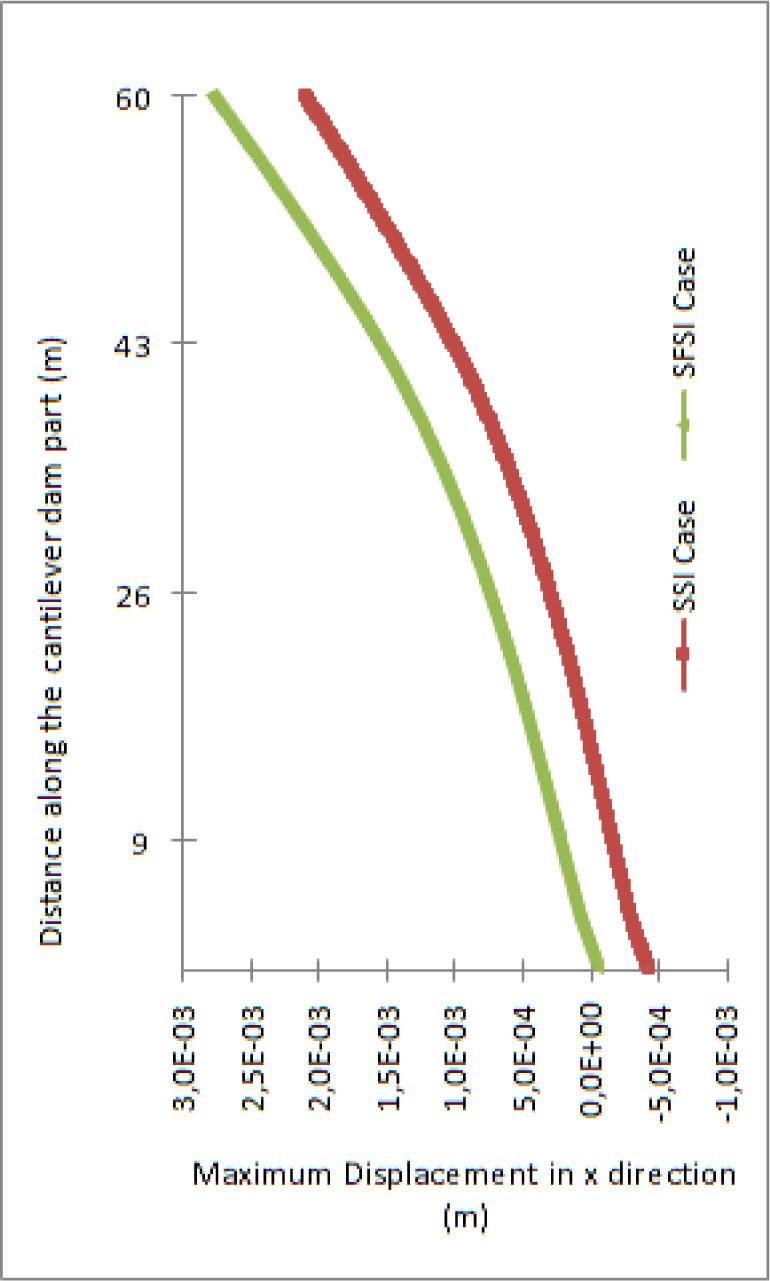Maximum displacement in X direction along the concrete dam cantilever path for the two studied cases

Figure 6 depicts the maximum displacement in the Y direction. The results show that the reservoir water presence has limited effects. The highest value, <0.02 mm, is very small due to very high stiffness along this direction.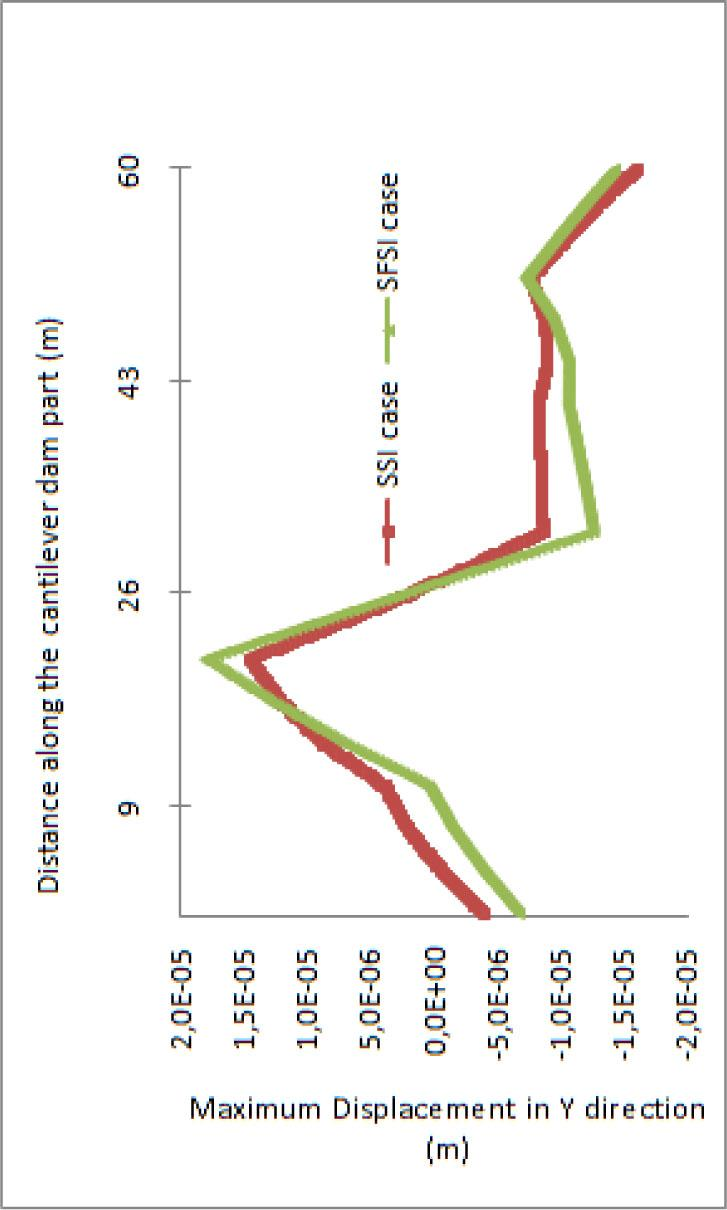Maximum displacement in the Y direction along the concrete dam cantilever path for the two studied cases

Figure 7 plots the maximum displacement in the Z direction along the concrete dam cantilever path. As shown, the effect of water is practically zero.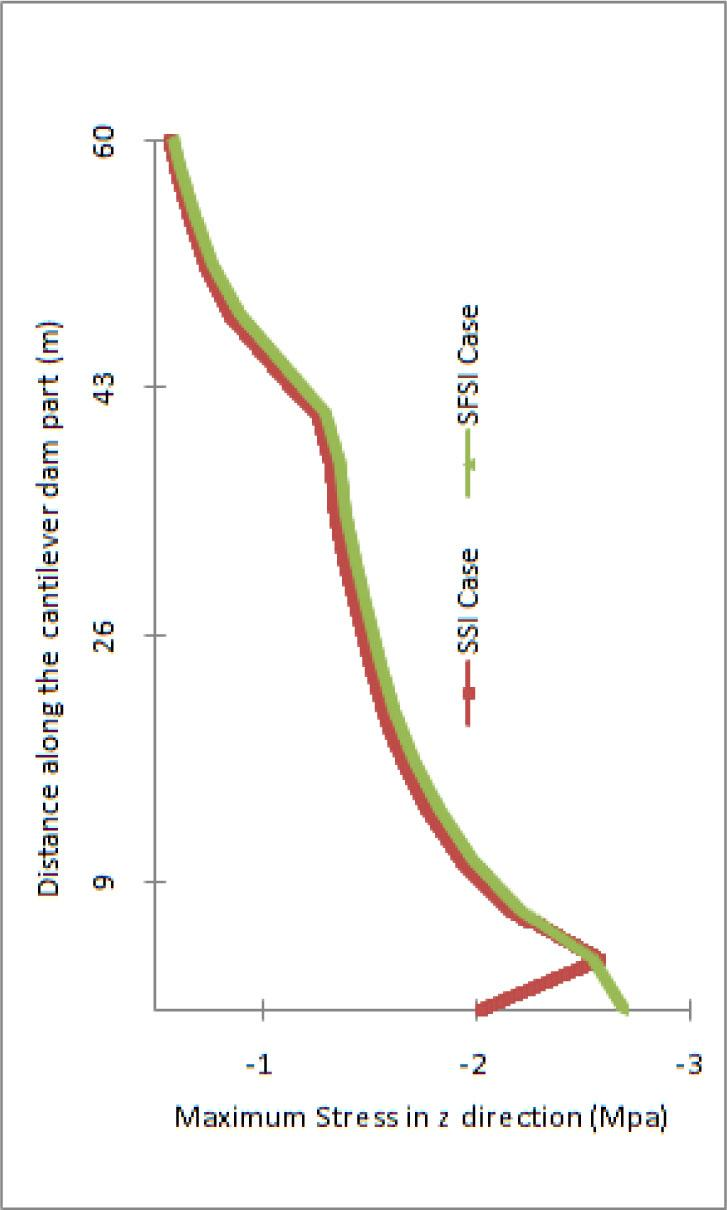Maximum displacement in the Z direction along the concrete dam cantilever path for the two studied cases

Figure 8 shows the effect of reservoir water on the maximum stress in the X direction along the concrete dam cantilever path. The effect of water is observed only in the vicinity of the foundation level, diminishing the magnitude. The range of the stress is smaller in the presence of reservoir water, compared to that in the absence of reservoir water.

Similarly, the effect of water presence on the maximum stress in the Y direction along the concrete dam cantilever path is depicted in Figure 9. As shown, the reservoir water presence increases this stress, but the highest magnitude, 0.27 MPa, is at a very low level.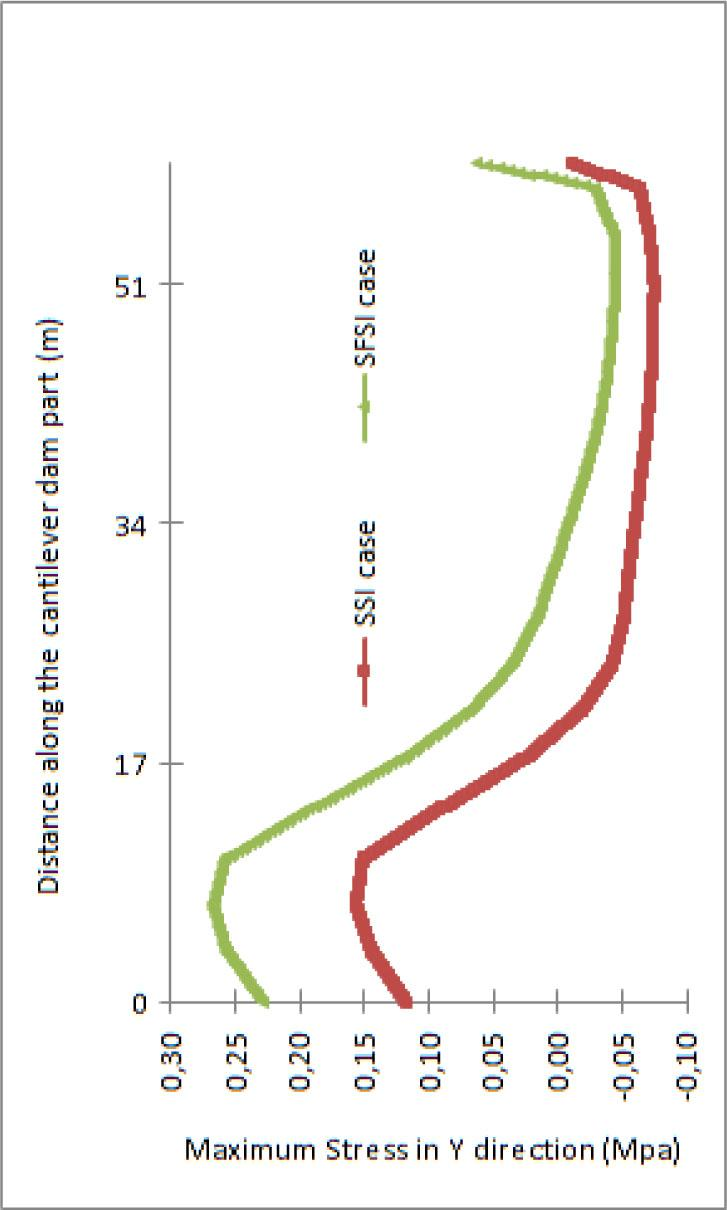Maximum stress in the Y direction along the concrete dam cantilever path for the two studied cases

Figure 10 plots the effect of water presence on the maximum stress in the Z direction along the concrete dam cantilever path. The influence of water is practically zero except in the vicinity of the foundation level, where an increase is observed.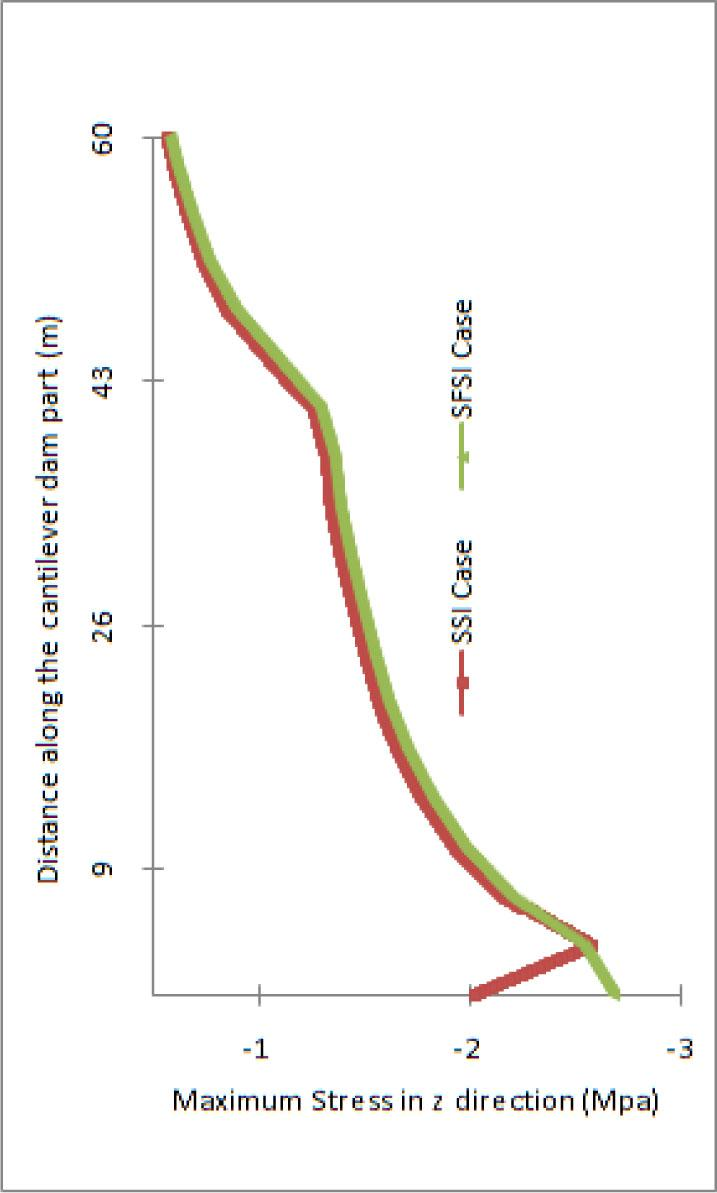Maximum stress in the Z direction along the concrete dam cantilever path for the two studied cases

Figure 11 shows the effect of water presence on maximum von Mises stress.

Figure 12 plots the maximum displacement in the X direction (radial direction) along the concrete dam crest path for the two studied cases (“concrete dam”–“foundation rock” interaction case [noted in the figure as “SSI case”] and “concrete dam”–“reservoir water”–“foundation rock” interaction case [noted in the figure as “SFSI case”]). As shown, the reservoir water presence significantly increases the displacement along the crest path, but the highest value, 3 mm, remains very small.

Similarly, Figure 13 depicts the maximum displacement in the Y direction (tangential/circumferential) along the concrete dam crest path. It can be seen from this figure that in the first half of the path, the increase in displacement due to reservoir water is slight, while the increase is more important in the second half.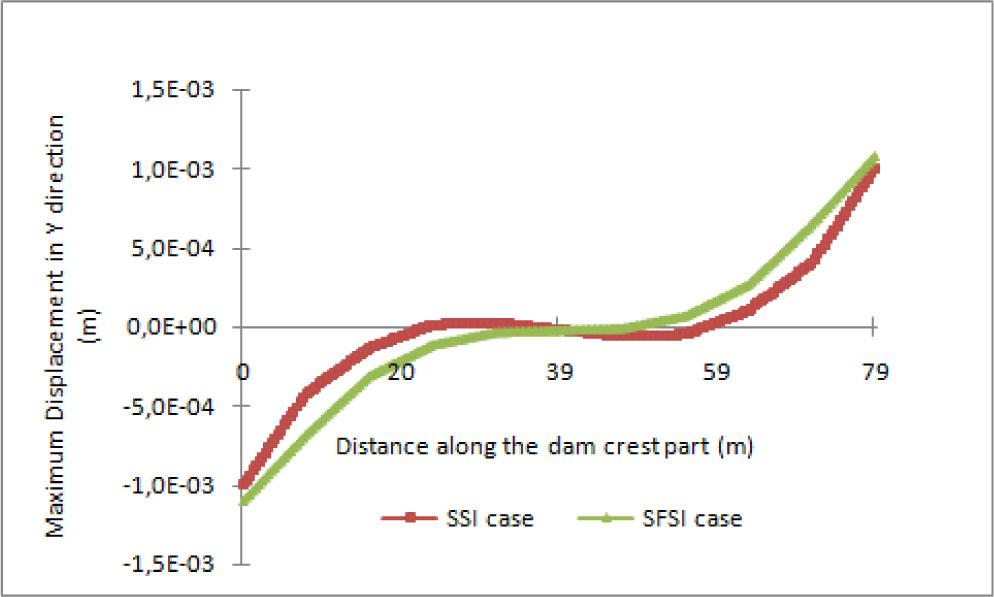Maximum displacement in the Y direction along the concrete dam crest path for the two studied cases

Figure 14 plots the maximum displacement in the Z direction along the concrete dam crest path. Clearly, reservoir water presence very significantly decreases the displacement, diminishing, for example, at the end of the crest path, from about −10 mm to 1 mm.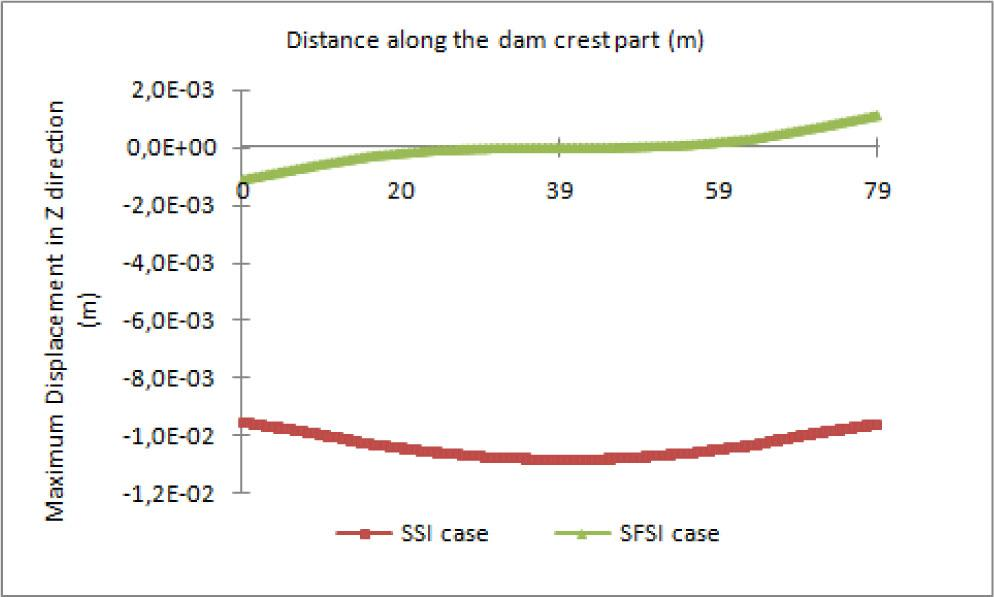Maximum displacement in the Z direction along the concrete dam crest path for the two studied cases

Figure 15 shows the effect of reservoir water presence on maximum stress in the X direction (radial direction along the concrete dam crest path). The presence of reservoir water very significantly decreases the stress magnitude at the locations near the two end points. The range of stress in the presence of reservoir water is notably smaller compared to that observed in its absence. However, the stress values in both the cases are very low.

Similarly, the effect of water presence on the maximum stress in the Y direction (tangential/circumferential direction) along the concrete dam crest path is depicted in Figure 16. Reservoir water decreases the stress magnitude in the first portion of the path, and then the effects are mixed. The influence of reservoir water here is mixed. Again, the range of stress observed in the presence of reservoir water is markedly smaller compared to that observed in its absence. Also, the stress values in both the cases are very low.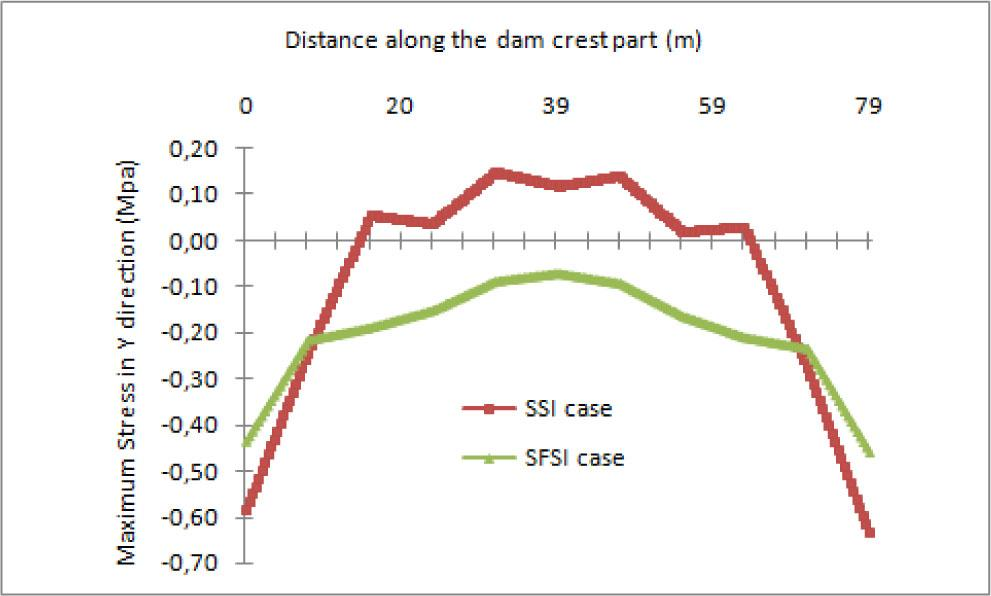The maximum stress in the Y direction (tangential/circumferential direction) along the concrete dam crest path for the two studied cases

Figure 17 plots the effect of reservoir water on the maximum stress in the Z direction along the concrete dam crest path. The influence is small except at the end part of the path, where an increase due to reservoir water presence can be seen. The highest value, at 1 MPa, is again very small.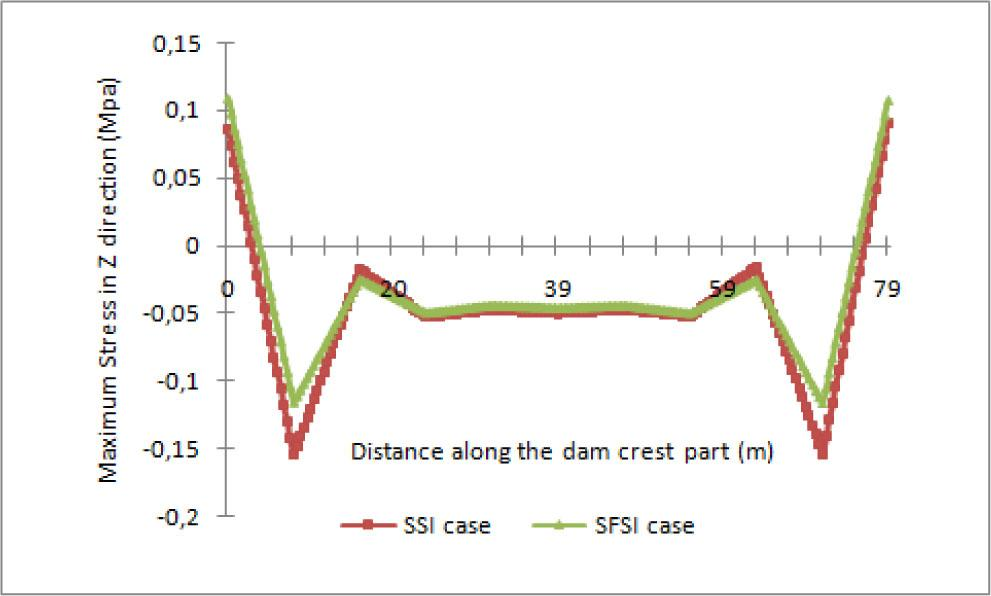The maximum stress in the Z direction along the concrete dam crest path for the two studied cases

Figure 18 shows the effect of reservoir water presence on the maximum von Mises stress along the crest path. As in Figure 17, the influence is small except at the end part of the path, where an increase due to reservoir water can be seen. The highest value, at 1 MPa, is very small.

Conclusions

This study presents the transient responses of the “Brezina concrete dam”–“reservoir water”–“mass rock–foundation” system using 3D Ansys finite element model. The dam is subjected to an acceleration seismic record applied in the X direction. The Lagrangian approach is used to model the reservoir water, and coupling equations are used to model the “reservoir water”–“concrete dam” and “reservoir water”–“rock foundation” interfaces. The effect of reservoir water on the displacements and stresses are analyzed along the cantilever path and the crest path of the concrete dam upstream face. The following conclusions are drawn based on the analyses carried out in this study.

Along the dam cantilever part:

Reservoir water increases the longitudinal displacement, the effects are negligible on the other displacement component(s), and the displacement magnitude is very small.

With or without reservoir water, the longitudinal and vertical stresses are in compression. For the 3D model, reservoir water diminishes (the influence is small) von Mises stress.

Along the dam crest part:

Reservoir water increases the longitudinal displacement, while it drastically diminishes the vertical displacement. The influence on the displacement along the crest direction is small. The displacement magnitude is also very small.

With or without reservoir water, the stress components are mostly in compression. Reservoir water is seen mostly to be diminishing the stresses, although, on the whole, its influence on von Mises stress is small. Again, the stress magnitude is very low.

Globally speaking, the role of reservoir water is mixed. In some cases, its presence is like a damper, which decreases the range of displacement or stresses, whereas in other cases, it increases or has a negligible effect on these quantities. Finally, it is worth emphasizing that the stresses determined are very low; thus, if the dam were to be subjected to a combination of three accelerations, it is expected that the resulting stress magnitude obtained would remain at a low or very low level.

#### Material properties of Brezina arch dam, foundation rock, and reservoir water

Material Young's modulus (N/m2) Poisson's ratio Density (kg/m3)
Concrete dam 28.5e+09 0.2 2,500
Foundation rock 14.5e+09 0.25 2,100
Reservoir water Compressibility modulus (N/m2) Viscosity 0.49 1,000
2.068E+09 0.001

#### Geometrical characteristics of Brezina dam

High (m) Arch length (m) Thickness (m)
60 78.5 At crest: 5
At foundation level: 36.3

#### ITS for the two dam models

Model type Fundamental frequency (Hz) ITS to resolve the response frequency (ITS = 1/20f) (s) ITS to resolve the applied load-versus-time curve (s) (ITS = 1/180f) (s)
3D full dam with mass foundation 7.566 0.0066 0.0007342

Ouzandja. D, Tiliouine. B, Belharizi. M. and Kadri. M. (2017). Three-dimensional nonlinear seismic response of oued fodda concrete gravity dam considering contact elements at dam-reservoir interaction interface. Asian journal of civil engineering (bhrc) vol. 18, no. 6, pages 977–992. OuzandjaD TiliouineB BelhariziM. KadriM. 2017 Three-dimensional nonlinear seismic response of oued fodda concrete gravity dam considering contact elements at dam-reservoir interaction interface Asian journal of civil engineering (bhrc) 18 6 977 992 Search in Google Scholar

Chopra Ak, Chakrabarti P. (1981). Earthquake analysis of concrete gravity dams including dam-water-foundation rock interaction, Earthquake Engineering & Structural Dynamics, No. 4, 9363–83. ChopraAk ChakrabartiP 1981 Earthquake analysis of concrete gravity dams including dam-water-foundation rock interaction Earthquake Engineering & Structural Dynamics 4 9363 83 10.1002/eqe.4290090406 Search in Google Scholar

Leger P, Boughoufalah M. (1989). Earthquake input mechanisms for time domain analysis of damfoundation systems, Engineering Structures, No. 1, 1137–46. LegerP BoughoufalahM. 1989 Earthquake input mechanisms for time domain analysis of damfoundation systems Engineering Structures 1 1137 46 Search in Google Scholar

Saleh S, Madabhushi SPG. (2010). Response of concrete dams on rigid and soil foundations under earthquake loading, Earthquake Tsunami, No. 3, 4251–68. SalehS MadabhushiSPG 2010 Response of concrete dams on rigid and soil foundations under earthquake loading Earthquake Tsunami 3 4251 68 10.1142/S1793431110000820 Search in Google Scholar

Lebon G, Saouma V, Uchita Y. (2010). 3D rock-dam seismic interaction, Dam Engineering, No. 2, 21101–30. LebonG SaoumaV UchitaY. 2010 3D rock-dam seismic interaction Dam Engineering 2 21101 30 Search in Google Scholar

Saouma V, Miura F, Lebon G, Yagome Y. (2011). A simplified 3D model for soil-structure interaction with radiation damping and free field input, Bulletin of Earthquake Engineering, No. 5, 91387–402. SaoumaV MiuraF LebonG YagomeY 2011 A simplified 3D model for soil-structure interaction with radiation damping and free field input Bulletin of Earthquake Engineering 5 91387 402 10.1007/s10518-011-9261-7 Search in Google Scholar

Burman A, Nayak P, Agrawal P, Maity D. (2012). Coupled gravity dam-foundation analysis using a simplified direct method of soil-structure interaction, Soil Dynamic Earthquake Engineering, No. 1, 3462–68. BurmanA NayakP AgrawalP MaityD 2012 Coupled gravity dam-foundation analysis using a simplified direct method of soil-structure interaction Soil Dynamic Earthquake Engineering 1 3462 68 10.1016/j.soildyn.2011.10.008 Search in Google Scholar

Hariri-Ardebili MA. (2014). Impact of foundation nonlinearity on the crack propagation of high concrete dams, Soil Mechanics and Foundation Engineering, No. 2, 5172–82. Hariri-ArdebiliMA 2014 Impact of foundation nonlinearity on the crack propagation of high concrete dams Soil Mechanics and Foundation Engineering 2 5172 82 10.1007/s11204-014-9257-9 Search in Google Scholar

Hatami K. (2008). Numerical simulation of dynamic soil-structure interaction in shaking table testing. Soil Dynamics and Earthquake Engineering; 28:453–467. HatamiK. 2008 Numerical simulation of dynamic soil-structure interaction in shaking table testing Soil Dynamics and Earthquake Engineering 28 453 467 10.1016/j.soildyn.2007.07.011 Search in Google Scholar

Fok KL, Chopra AK. (1986). Frequency response functions for arch dams: Hydrodynamic and foundation flexibility effects. Earthquake Engineering and Structural Dynamics; 14, 769–795. FokKL ChopraAK 1986 Frequency response functions for arch dams: Hydrodynamic and foundation flexibility effects Earthquake Engineering and Structural Dynamics 14 769 795 10.1002/eqe.4290140507 Search in Google Scholar

Tahar Berrabah A,(2013). Dynamic Soil-Fluid-Structure Interaction Applied For Concrete Dam, Doctorat Thesis, Tlemcen University, Algeria. Tahar BerrabahA 2013 Dynamic Soil-Fluid-Structure Interaction Applied For Concrete Dam Doctorat Thesis, Tlemcen University Algeria Search in Google Scholar

Bayraktar A, Hancer E, Akkose M. (2005). Influence of base-rock characteristics on the stochastic dynamic response of dam-reservoir-foundation systems. Engineering Structures; 27(10), 1498–1508. BayraktarA HancerE AkkoseM 2005 Influence of base-rock characteristics on the stochastic dynamic response of dam-reservoir-foundation systems Engineering Structures 27 10 1498 1508 10.1016/j.engstruct.2005.05.004 Search in Google Scholar

Tahar Berrabah A, Belharizi M, Laulusa A, Bekkouche A. (2012). Three-dimensional modal analysis of a concrete arch dam. Earth Science Research; 1(2), ISSN 1927-0542. Tahar BerrabahA BelhariziM LaulusaA BekkoucheA 2012 Three-dimensional modal analysis of a concrete arch dam Earth Science Research 1 2 ISSN 1927-0542. Search in Google Scholar

ANSYS. ANSYS User's Manual, ANSYS Theory Manual. Version 11.0, 2007. ANSYS ANSYS User's Manual, ANSYS Theory Manual. Version 11.0 2007 Search in Google Scholar

Blevins RD. (2001). Formulas for natural frequency and mode shape. Van Nostrand Reinhold Company, New York, Book. BlevinsRD 2001 Formulas for natural frequency and mode shape Van Nostrand Reinhold Company New York, Book Search in Google Scholar

Armouti, N. S. (2004). “Evaluation of Structural Response Subjected to Synthetic Earthquake Excitation”. Journal of Structural Engineering Vol. 31, 175–180. ArmoutiN. S. 2004 “Evaluation of Structural Response Subjected to Synthetic Earthquake Excitation” Journal of Structural Engineering 31 175 180 Search in Google Scholar

Tahar Berrabah A, Belharizi M, Laulusa A, Bekkouche A. (2015). Fluid–structure interaction of Brezina arch dam: 3D modal analysis. Engineering Structures; 84 19–28. Tahar BerrabahA BelhariziM LaulusaA BekkoucheA 2015 Fluid–structure interaction of Brezina arch dam: 3D modal analysis Engineering Structures 84 19 28 10.1016/j.engstruct.2014.11.011 Search in Google Scholar

Maeso O and Dominguez J. (1993). Earthquake analysis of arch dams. I. Dam-foundation interaction. J. Engineering Mechanics, ASCE; 119(3), 496–512. MaesoO DominguezJ 1993 Earthquake analysis of arch dams. I. Dam-foundation interaction J. Engineering Mechanics, ASCE 119 3 496 512 10.1061/(ASCE)0733-9399(1993)119:3(496) Search in Google Scholar

Tan H, Chopra AK. (1995). Dam-Foundation rock interaction effects in frequency response functions of arch dams, Earthquake Engineering and Structural Dynamics; 24, 1475–1489. TanH ChopraAK 1995 Dam-Foundation rock interaction effects in frequency response functions of arch dams Earthquake Engineering and Structural Dynamics 24 1475 1489 10.1002/eqe.4290241105 Search in Google Scholar

Sani AA, Lotfi V. (2010). Dynamic analysis of concrete arch dams by ideal-coupled modal approach. Engineering Structures; 32(5), 1377–1383. SaniAA LotfiV 2010 Dynamic analysis of concrete arch dams by ideal-coupled modal approach Engineering Structures 32 5 1377 1383 10.1016/j.engstruct.2010.01.016 Search in Google Scholar

Denghong Chen, Chunping Hou, and Feng Wang (2021). Influences on the Seismic Response of the Gravity Dam-Foundation-Reservoir System with Different Boundary and Input Models. Hindawi Shock and Vibration Volume 2021, Article ID 6660145, 15 pages. https://doi.org/10.1155/2021/6660145. ChenDenghong HouChunping WangFeng 2021 Influences on the Seismic Response of the Gravity Dam-Foundation-Reservoir System with Different Boundary and Input Models Hindawi Shock and Vibration 2021 Article ID 6660145, 15 pages. https://doi.org/10.1155/2021/6660145. 10.1155/2021/6660145 Search in Google Scholar

T. Menouillard, A. D. Tzenkov, and M. V. Schwager. (2021). Dynamic Analysis of Pine Flat Concrete Dam: Acoustic Fluid-Structural Interaction with ANSYS Workbench. https://www.researchgate.net/publication/344904885. MenouillardT. TzenkovA. D. SchwagerM. V. 2021 Dynamic Analysis of Pine Flat Concrete Dam: Acoustic Fluid-Structural Interaction with ANSYS Workbench https://www.researchgate.net/publication/344904885. Search in Google Scholar

Recommended articles from Trend MD